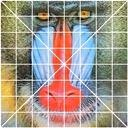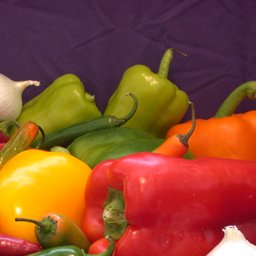## AUTOWHITE

 Automatically adjusts the white balance of an image.

### EXAMPLES

 Variation In Percent Pixels Used To Compute Average Original Image Arguments: -m 1 -p 0 Arguments: -m 1 -p 1 Arguments: -m 1 -p 5Original Image Arguments: -m 2 -p 0 Arguments: -m 2 -p 1 Arguments: -m 2 -p 5Comparison Method 1 And 2 --- Percent=1 Original Image Arguments: -m 1 -p 1 Arguments: -m 2 -p 1Comparison Method 1 And 2 --- Percent=1 Original Image Arguments: -m 1 -p 1 Arguments: -m 2 -p 1Comparison Method 1 And 2 --- Percent=1 Original Image Arguments: -m 1 -p 1 Arguments: -m 2 -p 1Comparison Method 1 And 2 --- Percent=1 Original Image Arguments: -m 1 -p 1 Arguments: -m 2 -p 1What the script does is as follows: Converts the image to RGB and separates channels Creates a mask from the negated saturation channel multiplied by the brightness channel from HSB so that the most intense 1% (default) pixels are white and the rest black Multiplies the mask image by each channel image Computes the mean of the mask image (maskmean) and the mean of each masked channel image (mean) Computes the ratio of maskmean/mean for each channel Applies -recolor to the original image using the ratios for the main diagonal elements of the matrix and zero elsewhere This is equivalent to the following IM commands for method=1. convert \$infile -colorspace RGB -channel R -separate \$tmpR1 convert \$infile -colorspace RGB -channel G -separate \$tmpG1 convert \$infile -colorspace RGB -channel B -separate \$tmpB1 convert \$tmpA1 -colorspace HSB -channel G -negate -channel GB -separate +channel \ -compose multiply -composite +channel \ -contrast-stretch 0,\${percent}% -fill black +opaque white \ \$tmpM1 maskmean=`convert \$tmpM1 -format "%[mean]" info:` convert \$tmpR1 \$tmpM1 -compose multiply -composite \$tmpT1 mean=`convert \$tmpT1 -format "%[mean]" info:` redratio=`convert xc: -format "%[fx:\$maskmean/\$mean]" info:` convert \$tmpG1 \$tmpM1 -compose multiply -composite \$tmpT1 mean=`convert \$tmpT1 -format "%[mean]" info:` greenratio=`convert xc: -format "%[fx:\$maskmean/\$mean]" info:` convert \$tmpB1 \$tmpM1 -compose multiply -composite \$tmpT1 mean=`convert \$tmpT1 -format "%[mean]" info:` blueratio=`convert xc: -format "%[fx:\$maskmean/\$mean]" info:` convert \$tmpA1 -recolor "\$redratio 0 0 0 \$greenratio 0 0 0 \$blueratio" \$outfile